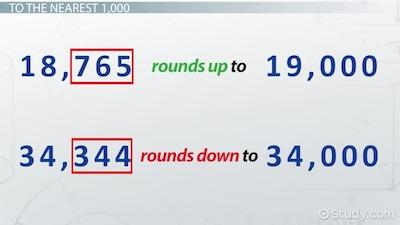# Rounding Numbers – Hardware Implementations And Implications

Rounding numbers is a part of our daily lives. However, it is important to acknowledge that rounding numbers may have some implications especially when you are performing operations.

Learn how to round numbers up and down.

However, before we get into that, it’s important to revise a couple of concepts such as the differences between integer, fractional, fixed-point, and floating-point values.

## Fixed-Point

Simply put, fixed-point refers to a way of writing, representing, or storing in the case of computers, numerical quantities with a predetermined number of digits and with the “point” located at a single, unchanging position. In the case of decimals, this would be the decimal point, for example. In the case of binary numbers, it would be the binary point.

For example, a fixed-point sign-magnitude decimal number supporting three digits to the left of the decimal point and two digits to the right could be used to represent values in the range “999.99 to +999.99. From this, you realize that integer representations are a special case of fixed-point in which there are no fractional digits. Similarly, purely fractional representations are a special case of fixed-point in which there are no integer digits.

## Floating-Point

The floating-point is just the opposite of the fixed-point. After all, it’s a value that is expressed as a multiple of an appropriate power of the base of the number system. This means the point can move around.

For example, using floating-point notation, the value 12.34 can also be written as 123.4 × 10^-1, 1234 × 10^-2, 12340 × 10^-3, and so forth; and also as 1.234 × 10^^1, 0.1234 × 10^2, 0.01234 × 10^3, and so forth.

## Converting From Floating-Point To Fixed-Point

One very common case where rounding proves necessary is when converting from floating-point representations into their fixed-point counterparts. For example, DSP algorithms are usually first analyzed and evaluated using floating-point representations. These algorithms are subsequently recast into fixed-point representations for implementation in hardware.

Discover how to round off whole numbers.

In this case, it is possible to experience what are known as quantization errors or computational noise (where the term “quantization” refers to the act of limiting the possible values of some quantity or magnitude into a discrete set of values or quanta.)

When we come to hardware implementations of rounding algorithms, the most common techniques are truncation, round-half-up (which is commonly referred to as round-to-nearest), and round-floor (rounding towards negative infinity).

In the case of unsigned binary representations, truncation, round-half-up, and round-floor work as discussed above. However, there are some interesting nuances when it comes to signed binary representations.

Purely for the sake of discussion, let’s consider an 8-bit signed binary fixed-point representation comprising four integer bits and four fractional bits.

To make things simple, let’s assume that you wish to perform your various rounding algorithms so as to be left with only an integer value. Imagine that your 8-bit field contains a value of 0011.1000, which equates to +3.5 in decimal.

In the case of a truncation (or chopping) algorithm, we will simply discard the fractional bits, resulting in an integer value of 0011; this equates to 3 in decimal, which is what we would expect.

This is how to round percentages.

Next, assume a value of 1100.1000. The integer portion of this equates to -4, while the fractional portion equates to +0.5, resulting in a total value of -3.5. Thus, when we perform a truncation and discard our fractional bits, we end up with an integer value of 1100, which equates to -4 in decimal. Thus, in the case of a signed binary value, performing a truncation operation actually results in implementing a round-floor algorithm.